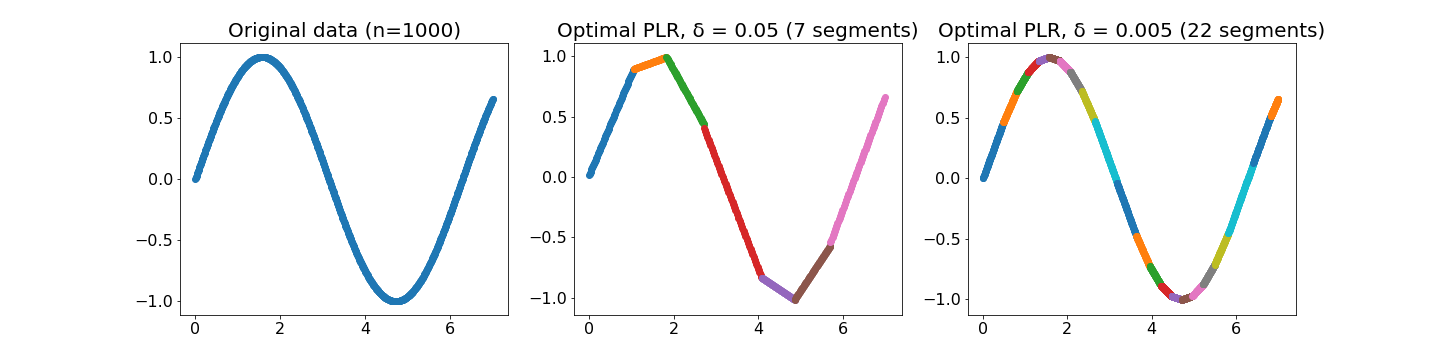# Crate plr

Expand description

This crate provides code for performing error-bounded piecewise linear regression (PLR) in an online fashion using either a greedy (constant time per point, constant space) or optimal (linear time per point, linear space) algorithm. Both algorithms were implemented as described in:

Qing Xie, Chaoyi Pang, Xiaofang Zhou, Xiangliang Zhang, and Ke Deng. 2014. Maximum error-bounded Piecewise Linear Representation for online stream approximation. The VLDB Journal 23, 6 (December 2014), 915–937. DOI: https://doi.org/10.1007/s00778-014-0355-0

Error-bounded piecewise linear regression is the task of taking a set of datapoints and finding a piecewise linear function that approximates each datapoint within a fixed bound. In other words, given a dataset `(x, y) ∈ D`, we seek a piecewise linear function `f` such that `∀(x, y)∈D(|f(x) - y| < δ)`, for some given δ. This can also be thought of as minimizing the “L-infinity” loss. Since `f` could be trivially realized by a piecewise linear function with `|D|` pieces, we also seek the `f` with a minimal number of pieces.In the example above, we show the original 1000 data points (left), a PLR with a maximum error of 0.05 (center), and a PLR with a maximum error of 0.005. All of the displayed PLRs are optimal, meaning that they contain the fewest number segments possible to achieve their error bound.

This package can perform PLR using one of two online algorithms:

• Greedy PLR, which uses a constant time per point and a constant amount of space. Greedy PLR always finds a PLR with the specified error bound (e.g., none of the original points will be more than δ from their predictions), but the greedy approach may not find the PLR with the minimal number of segments.
• Optimal PLR, which uses a linear time per point and potentially linear space. In practice, the amount of space used by the optimal algorithm should be small, but in the worst case linear space may be required (see the paper for more details). The optimal algorithm will find the solution with a minimal number of segments.

This package can also perform spline regression instead of piecewise linear regression. See greedy_splines, or the online version GreedySpline.

Written by Ryan Marcus, licensed under the GPL v3.

## Structs

A single segment of a PLR. The `start` field is inclusive, the `stop` field is exclusive. The `slope` and `intercept` field can be used in a linear model: in other words, for some `x` such that `start <= x < stop`, the prediction is `slope * x + intercept`.